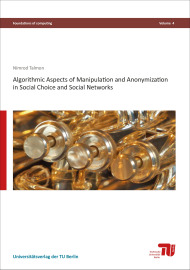# Algorithmic Aspects of Manipulation and Anonymization in Social Choice and Social NetworksSize: 289 pages
Format: 14,8 x 21,0 cm
Publishing year: 2016
ISBN 978-3-7983-2804-4
13,00

This thesis presents a study of several combinatorial problems related to social choice and social networks. The main concern is their computational complexity, with an emphasis on their parameterized complexity. The goal is to devise efficient algorithms for each of the problems studied here, or to prove that, under widely-accepted assumptions, such algorithms cannot exist.
The problems discussed in Chapter 3 and in Chapter 4 are about manipulating a given election, where some relationships between the entities of the election are assumed. This can be seen as if the election occurs on top of an underlying social network, connecting the voters participating in the election or the candidates which the voters vote on.
The problem discussed in Chapter 3, Combinatorial Candidate Control, is about manipulating an election by changing the set of candidates which the voters vote on. That is, there is an external agent who can add new candidates or delete existing candidates. A combinatorial structure over the candidates is assumed, such that whenever the external agent adds or removes a candidate, a predefined set of candidates (related to the chosen candidate) are added or removed from the election.
The problem discussed in Chapter 4, Combinatorial Shift Bribery, is also about manipulating an election. Here, however, the external agent can change the way some voters vote. Specifically, a combinatorial structure over the voters is assumed, such that the external agent can change the position of its preferred candidate in sets of voters, following some predefined patterns.
The problem discussed in Chapter 5, Election Anonymization, is also about elections. The main concern here, however, is preserving the privacy of the voters, when the votes are published, along with some additional (private) information.
The task is to transform a given election such that each vote would appear at least k times. By doing so, even an adversary which knows how some voters vote, cannot identify individual voters.
The problems discussed in Chapter 6 and in Chapter 7 are also about privacy. Specifically, a social network (modeled as a graph) is to become publicly available. The task is to anonymize the graph; that is, to transform the graph such that, for every vertex, there will be at least \$k – 1\$ other vertices with the same degree. By doing so, even an adversary which knows the degrees of some vertices cannot identify individual vertices.
In the problem discussed in Chapter 6, Degree Anonymization by Vertex Addition, the way to achieve anonymity is by introducing new vertices.
In the problem discussed in Chapter 7, Degree Anonymization By Graph Contractions, the way to achieve anonymity is by contracting as few edges as possible.
The main aim of this thesis, considering the problems mentioned above, is to explore some boundaries between tractability and intractability. Specifically, as most of these problems are computationally intractable (that is, NP-hard or even hard to approximate), some restricted cases and parameterizations for these problems are considered.
The goal is to devise efficient algorithms for them, running in polynomial-time when some parameters are assumed to be constant, or, even better, to show that the problems are fixed-parameter tractable for the parameters considered.
If such algorithms cannot be devised, then the goal is to prove that these problems are indeed not fixed-parameter tractable with respect to some parameters, or, even better, to show that the problems are NP-hard even when some parameters are assumed to be constant.